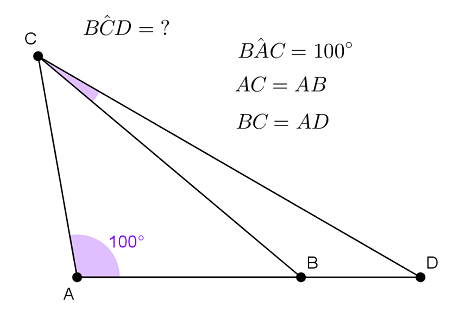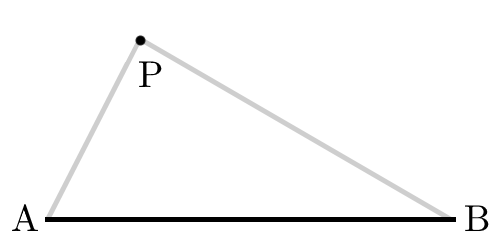Geometry

# Classification of Triangles: Level 3 ChallengesIn triangle $CAD$ we have $\hat { CAD } = 100°$. Point $B$ in on $AD$ such that $AC = AB$ and $BC = AD$. Find $\hat { BCD }$ in degrees.

For some value $d,$ an isosceles triangle has at least one angle of $(2d-40)$ degrees and at least one angle of $(d+20)$ degrees.

For each of such triangles, let $\alpha$ denote the smallest angle in degrees. Then what is the sum of all possible $\alpha$'s?

The sides of a triangle have lengths $11 , 15 , k$ where $k$ is an integer. For how many value(s) of $k$ is the triangle obtuse?

###### This question is a part of JEE Novices.

A triangle has sides of length $\sin( x), \cos (x)$ and $\sqrt{1+\sin (x )\cos( x)}$, with $x$ an acute angle.

Find the largest angle of the triangle in degrees.A line $AB$ of length $l$ makes up one side of a triangle. The locus of all points $P$ such that $\triangle ABP$ is a right triangle with $\angle P = 90^{\circ}$ encloses an area of $\text{\_\_\_\_\_\_\_\_\_\_}$.

The two larger angles of an isosceles triangle have a combined measure of $135^\circ$. If this triangle has a perpendicular height of $2\text{ m}$, find the length of its base in meters.

If the latter can be expressed in the form $a\sqrt { b } -c$, where $a,b$ and $c$ are positive integers with $b$ square-free, write your answer as $a\times b\times c$.

×